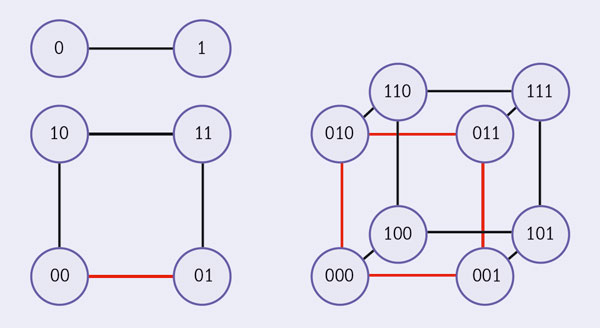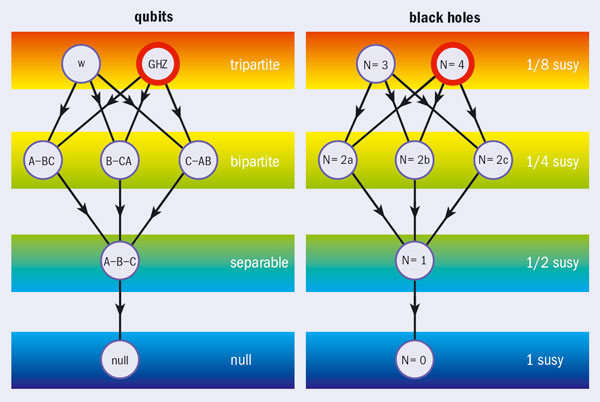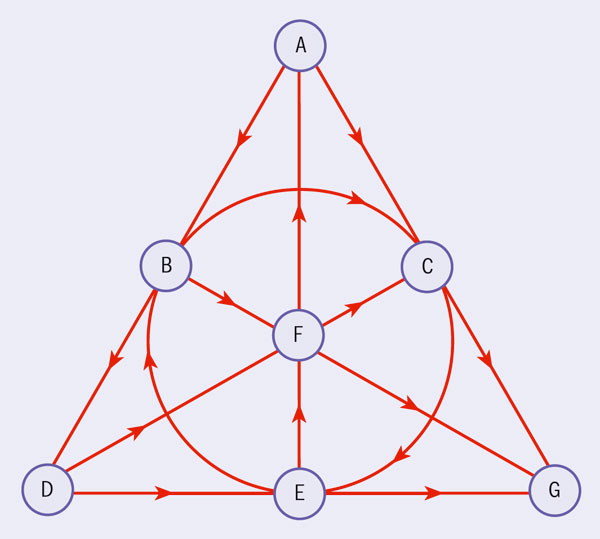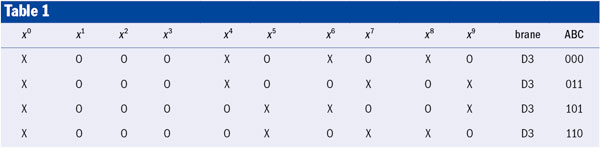# Black holes and qubits

5 May 2010

Michael Duff explains how string theory and M-theory could have practical applications in quantum information theory.Fig. 1. A single qubit is represented by a line, two qubits by a square and three qubits by a cube.

Quantum entanglement lies at the heart of quantum information theory (QIT), with applications to quantum computing, teleportation, cryptography and communication. In the apparently separate world of quantum gravity, the Hawking effect of radiating black holes has also occupied centre stage. Despite their apparent differences it turns out that there is a correspondence between the two (Duff 2007; Kallosh and Linde 2006).

Whenever two disparate areas of theoretical physics are found to share the same mathematics, it frequently leads to new insights on both sides. Indeed, this correspondence turned out to be the tip of an iceberg: knowledge of string theory and M-theory leads to new discoveries about QIT, and vice versa.

### Bekenstein-Hawking entropy

Every object, such as a star, has a critical size that is determined by its mass, which is called the Schwarzschild radius. A black hole is any object smaller than this. Once something falls inside the Schwarzschild radius, it can never escape. This boundary in space–time is called the event horizon. So the classical picture of a black hole is that of a compact object whose gravitational field is so strong that nothing – not even light – can escape.

Yet in 1974 Stephen Hawking showed that quantum black holes are not entirely black but may radiate energy. In that case, they must possess the thermodynamic quantity called entropy. Entropy is a measure of how disorganized a system is and, according to the second law of thermodynamics, it can never decrease. Noting that the area of a black hole’s event horizon can never decrease, Jacob Bekenstein had earlier suggested such a thermodynamic interpretation implying that black holes must have entropy. This Bekenstein–Hawking black-hole entropy is given by one quarter of the area of the event horizon.

Entropy also has a statistical interpretation as a measure of the number of quantum states available. However, it was not until 20 years later that string theory provided a microscopic explanation of this kind for black holes.

### Bits and pieces

A bit in the classical sense is the basic unit of computer information and takes the value of either 0 or 1. A light switch provides a good analogy; it can either be off, denoted 0, or on, denoted 1. A quantum bit or “qubit” can also have two states but whereas a classical bit is either 0 or 1, a qubit can be both 0 and 1 until we make a measurement. In quantum mechanics, this is called a superposition of states. When we actually perform a measurement, we will find either 0 or 1 but we cannot predict with certainty what the outcome will be; the best we can do is to assign a probability to each outcome.

There are many different ways to realize a qubit physically. Elementary particles can carry an intrinsic spin. So one example of a qubit would be a superposition of an electron with spin up, denoted 0, and an electron with spin down, denoted 1. Another example of a qubit would be the superposition of the left and right polarizations of a photon. So a single qubit state, usually called Alice, is a superposition of Alice-spin-up 0 and Alice-spin-down 1, represented by the line in figure 1. The most general two-qubit state, Alice and Bob, is a superposition of Alice-spin-up–Bob-spin-up 00, Alice-spin-up–Bob-spin-down 01, Alice-spin-down–Bob-spin-up 10 and Alice-spin-down–Bob-spin-down 11, represented by the square in figure 1.

Consider a special two-qubit state that is just 00 + 01. Alice can only measure spin up but Bob can measure either spin up or spin down. This is called a separable state; Bob’s measurement is uncorrelated with that of Alice. By contrast, consider 00 + 11. If Alice measures spin up, so too must Bob, and if she measures spin down so must he. This is called an entangled state; Bob cannot help making the same measurement. Mathematically, the square in figure 1 forms a 2 × 2 matrix and a state is entangled if the matrix has a nonzero determinant.

This is the origin of the famous Einstein–Podolsky–Rosen (EPR) paradox put forward in 1935. Even if Alice is in Geneva and Bob is millions of miles away in Alpha Centauri, Bob’s measurement will still be determined by that of Alice. No wonder Albert Einstein called it “spooky action at a distance”. EPR concluded rightly that if quantum mechanics is correct then nature is nonlocal, and if we insist on local “realism” then quantum mechanics must be incomplete. Einstein himself favoured the latter hypothesis. However, it was not until 1964 that CERN theorist John Bell proposed an experiment that could decide which version was correct – and it was not until 1982 that Alain Aspect actually performed the experiment. Quantum mechanics was right, Einstein was wrong and local realism went out the window. As QIT developed, the impact of entanglement went far beyond the testing of the conceptual foundations of quantum mechanics. Entanglement is now essential to numerous quantum-information tasks such as quantum cryptography, teleportation and quantum computation.

### Cayley’s hyperdeterminant

As a high-energy theorist involved in research on quantum gravity, string theory and M-theory, I paid little attention to any of this, even though, as a member of staff at CERN in the 1980s, my office was just down the hall from Bell’s.

My interest was not aroused until 2006, when I attended a lecture by Hungarian physicist Peter Levay at a conference in Tasmania. He was talking about three qubits Alice, Bob and Charlie where we have eight possibilities,000 , 001, 010, 011, 100, 101, 110, 111, represented by the cube in figure 1. Wolfgang Dür and colleagues at the University of Innsbruck have shown that three qubits can be entangled in several physically distinct ways: tripartite GHZ (Greenberger–Horne–Zeilinger), tripartite W, biseparable A-BC, separable A-B-C and null, as shown in the left hand diagram of figure 2 (Dür et al. 2000).Fig. 2. The classification of three qubits (left) exactly matches the classification of black holes from N wrapped branes (right). Only the GHZ state has a nonzero 3-tangle and only the N = 4 black hole has nonzero entropy.

The GHZ state is distinguished by a nonzero quantity known as the 3-tangle, which measures genuine tripartite entanglement. Mathematically, the cube in figure 1 forms what in 1845 the mathematician Arthur Cayley called a “2 × 2 × 2 hypermatrix” and the 3-tangle is given by the generalization of a determinant called Cayley’s hyperdeterminant.

The reason this sparked my interest was that Levay’s equations reminded me of some work I had been doing on a completely different topic in the mid-1990s with my collaborators Joachim Rahmfeld and Jim Liu (Duff et al. 1996). We found a particular black-hole solution that carries eight charges (four electric and four magnetic) and involves three fields called S, T and U. When I got back to London from Tasmania I checked my old notes and asked what would happen if I identified S, T and U with Alice, Bob and Charlie so that the eight black-hole charges were identified with the eight numbers that fix the three-qubit state. I was pleasantly surprised to find that the Bekenstein–Hawking entropy of the black holes was given by the 3-tangle: both were described by Cayley’s hyperdeterminant.

### Octonions and super qubits

According to supersymmetry, for each known boson (integer spin 0, 1, 2 and so on) there is a fermion (half-integer spin 1/2, 3 /2, 5/2 and so on), and vice versa. CERN’s Large Hadron Collider will be looking for these superparticles. The number of supersymmetries is denoted by N and ranges from 1 to 8 in four space–time dimensions.

CERN’s Sergio Ferrara and I have extended the STU model example, which has N = 2, to the most general case of black holes in N = 8 supergravity. We have shown that the corresponding system in quantum-information theory is that of seven qubits (Alice, Bob, Charlie, Daisy, Emma, Fred and George), undergoing at most a tripartite entanglement of a specific kind as depicted by the Fano plane of figure 3.Fig. 3. The Fano plane. The vertices A, B, C, D, E, F, G represents the seven qubits, and the seven lines ABD, BCE, CDF, DEG, EFA, FGB, GAC represent the tripartite entanglement.

The Fano plane has a strange mathematical property: it describes the multiplication table of a particular kind of number: the octonion. Mathematicians classify numbers into four types: real numbers, complex numbers (with one imaginary part A), quaternions (with three imaginary parts A, B, D) and octonions (with seven imaginary parts A, B, C, D, E, F, G). Quaternions are noncommutative because AB does not equal BA. Octonions are both noncommutative and nonassociative because (AB)C does not equal A(BC).

Real, complex and quaternion numbers show up in many physical contexts. Quantum mechanics, for example, is based on complex numbers and Pauli’s electron-spin operators are quaternionic. Octonions have fascinated mathematicians and physicists for decades but have yet to find any physical application. In recent books, both Roger Penrose and Ray Streater have characterized octonions as one of the great “lost causes” in physics. So we hope that the tripartite entanglement of seven qubits (which is just at the limit of what can be reached experimentally) will prove them wrong and provide a way of seeing the effects of octonions in the laboratory (Duff and Ferrara 2007; Borsten et al. 2009a).

In another development, QIT has been extended to super-QIT with the introduction of the superqubit, which can take on three values: 0 or 1 or $. Here 0 and 1 are “bosonic” and$ is “fermionic” (Borsten et al. 2009b). Such values can be realized in condensed-matter physics, such as the excitations of the t-J model of strongly correlated electrons, known as spinons and holons. The superqubits promise totally new effects. For example, despite appearances, the two-superqubit state  is entangled. Superquantum computing is already being investigated (Castellani et al. 2010).

### Strings, branes and M-theory

If current ideas are correct, a unified theory of all physical phenomena will require some radical ingredients in addition to supersymmetry. For example, there should be extra dimensions: supersymmetry places an upper limit of 11 on the dimension of space–time. The kind of real, four-dimensional world that supergravity ultimately predicts depends on how the extra seven dimensions are rolled up, in a way suggested by Oskar Kaluza and Theodor Klein in the 1920s. In 1984, however, 11-dimensional supergravity was knocked off its pedestal by superstring theory in 10 dimensions. There were five competing theories: the E8 × E8 heterotic, the SO(32) heterotic, the SO(32) Type I, and the Type IIA and Type IIB strings. The E8 × E8 seemed – at least in principle – capable of explaining the elementary particles and forces, including their handedness. Moreover, strings seemed to provide a theory of gravity that is consistent with quantum effects.

However, the space–time of 11 dimensions allows for a membrane, which may take the form of a bubble or a two-dimensional sheet. In 1987 Paul Howe, Takeo Inami, Kelly Stelle and I showed that if one of the 11 dimensions were a circle, we could wrap the sheet round it once, pasting the edges together to form a tube. If the radius becomes sufficiently small, the rolled-up membrane ends up looking like a string in 10 dimensions; it yields precisely the Type IIA superstring. In a landmark talk at the University of Southern California in 1995, Ed Witten drew together all of this work on strings, branes and 11 dimensions under the umbrella of M-theory in 11 dimensions. Branes now occupy centre stage as the microscopic constituents of M-theory, as the higher-dimensional progenitors of black holes and as entire universes in their own right.

Such breakthroughs have led to a new interpretation of black holes as intersecting black-branes wrapped round the seven curled dimensions of M-theory or six of string theory. Moreover, the microscopic origin of the Bekenstein-Hawking entropy is now demystified. Using Polchinski’s D-branes, Andrew Strominger and Cumrun Vafa were able to count the number of quantum states of these wrapped branes (Strominger and Vafa 1996). A p-dimensional D-brane (or Dp-brane) wrapped round some number p of the compact directions (x4, x5, x6, x7, x8, x9) looks like a black hole (or D0-brane) from the four-dimensional (x0, x1, x2, x3) perspective. Strominger and Vafa found an entropy that agrees with Hawking’s prediction, placing another feather in the cap of M-theory. Yet despite all of these successes, physicists are glimpsing only small corners of M-theory; the big picture is still lacking. Over the next few years we hope to discover what M-theory really is. Understanding black holes will be an essential prerequisite.

### Falsifiable predictions?

The partial nature of our understanding of string/M-theory has so far prevented any kind of smoking-gun experimental test. This has led some critics of string theory to suggest that it is not true science. This is easily refuted by studying the history of scientific discovery; the 30-year time lag between the EPR idea and Bell’s falsifiable prediction provides a nice example (see Further reading). Nevertheless it cannot be denied that such a prediction in string theory would be welcome.Table 1. A GHZ state corresponds to a four-charge black hole in 4-dimensional space–time x0, x1, x2, x3 coming from four D3-branes each wrapping three of the six extra dimensions (x4, x6, x8), (x4, x7, x9), (x5, x6, x9) and (x5, x7, x8).

In string literature one may find D-brane intersection rules that tell us how N branes can intersect over one another and the fraction of supersymmetry (susy) that they preserve (Bergshoeff et al. 1997). In our black hole/qubit correspondence, my students Leron Borsten, Duminda Dahanayake, Hajar Ebrahim, William Rubens and I showed that the microscopic description of the GHZ state,000 +011+101+110 is that of the N = 4;1/8 susy case of D3-branes of Type IIB string theory (Borsten et al. 2008). We denoted the wrapped circles by crosses and the unwrapped circles by noughts; O corresponds to XO and 1 to OX, as in table 1. So the number of qubits here is three because the number of extra dimensions is six. This also explains where the two-valuedness enters on the black-hole side. To wrap or not to wrap; that is the qubit.

Repeating the exercise for the N <4 cases and using our dictionary, we see that string theory predicts the three-qubit entanglement classification of figure 2, which is in complete agreement with the standard results of QIT. Allowing for different p-branes wrapping different dimensions, we can also describe “qutrits” (three-state systems) and more generally “qudits” (d-state systems). Furthermore, for the well documented cases of 2 × 2, 2 × 3, 3 × 3, 2 × 2 × 3 and 2 × 2 × 4, our D-brane intersection rules are also in complete agreement. However, for higher entanglements, such as 2 × 2 × 2 × 2, the QIT results are partial or not known, or else contradictory. This is currently an active area of research in QIT because the experimentalists can now control entanglement with a greater number of qubits. One of our goals is to use the allowed wrapping configurations and D-brane intersection rules to predict new qubit-entanglement classifications.

So the esoteric mathematics of string and M-theory might yet find practical applications.

E Bergshoeff et al. 1997 Nucl. Phys. B494 119, arXiv:hep-th/ 9612095.

L Borsten et al. 2008 Phys. Rev. Lett. 100 251602, arXiv:0802.0840 [hep-th].

L Borsten et al. 2009a Phys. Rep. 471 113, arXiv:0809.4685 [hep-th].

L Borsten et al. 2009b arXiv:0908.0706 [quant-ph].

L Castellani et al. 2010 arXiv:1001.3753 [hep-th].

M J Duff et al. 1996 Nucl. Phys. B459 125, arXiv:hep-th/9508094.

M J Duff 2007 Phys. Rev. D76 025017, arXiv:hep-th/0601134.

M J Duff and S Ferrara 2007 Phys. Rev. D76 025018, arXiv:quant-ph /0609227.

W Dür et al. 2000 Phys. Rev. A62 062314, arXiv:quant-ph /0005115.

R Kallosh and A Linde 2006 Phys. Rev. D73 104033, arXiv:hep-th /0602061.

A Strominger and C Vafa 1996 Phys. Lett. B379 99, arXiv:hep-th /9601029.

### Events

• Theory | School TASI 2023 5—30 June 2023 | Boulder, US
• Theory | Conference SUSY 2023 17—21 July 2023 | Southampton, UK
• Theory | Symposium Lattice 2023 31 July — 4 August 2023 | Batavia, US